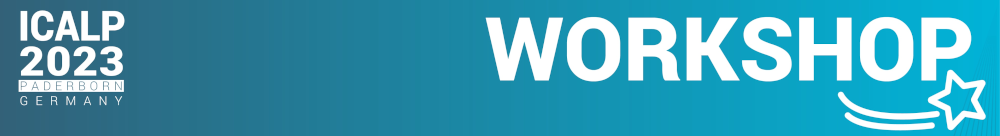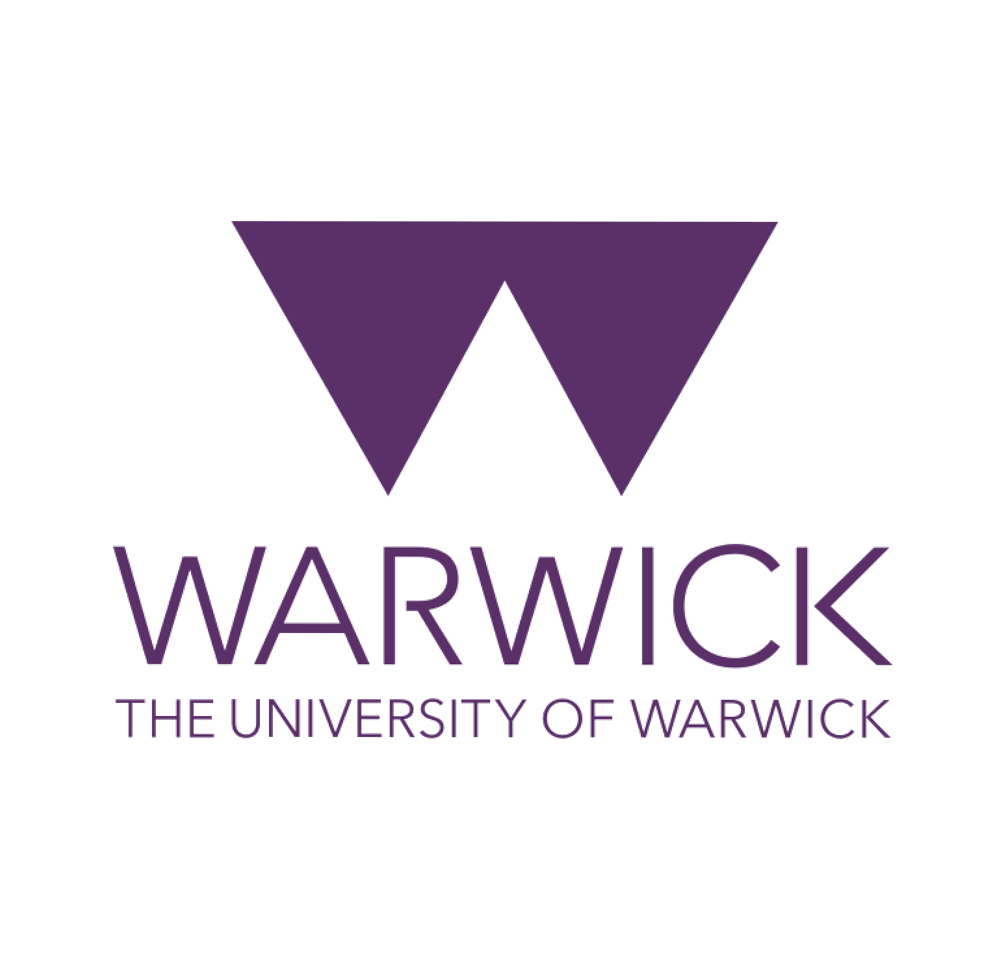# Algebraic Complexity Theory Workshop at ICALP 2023## Time and Place

The aim of this 1-day satellite workshop of ICALP 2023 is to initiate interactions and collaborations between the algebraic complexity theory community and the wider theoretical computer science community.

Algebraic Complexity Theory is a vibrant field that has seen a tremendous amount of activity in the recent years. Its classical questions have been interwoven with deep questions from algebraic geometry, invariant theory, and representation theory. Researchers study a wide range of interlinked topics: arithmetic circuit lower bounds, algorithmic algebra(ic geometry), algorithmic invariant theory, geometric complexity theory, tensor rank, polynomial identity testing, and polynomial reconstruction, to name a few.

The presentations are aimed at a general theoretical computer science audience.

## Plenary Speakers

• Hervé Fournier (Université de Paris, Institut de Mathématiques de Jussieu-Paris Rive Gauche)

## Schedule

The workshop is embedded into the official ICALP schedule.

## Abstracts (sorted alphabetically by last name)

• Prashanth Amireddy [slides]
A survey of algebraic circuit lower bounds
Proving algebraic circuit lower bounds is a prerequisite to and supposedly easier than proving P \ne NP. However, until recently, we did not even have superpolynomial lower bounds for constant-depth algebraic circuits. In this talk, I will discuss this breakthrough result and its surprisingly simple proof(s). I will also talk about a few other incomparable lower bound results for restricted models and argue how proving "good" lower bounds, even for these restricted models, implies general circuit/formula lower bounds. I will conclude by pointing to some common themes across these proofs and state some open problems.
• Pranjal Dutta [slides]
Polynomial Factorization: Recent advances, and challenges
Polynomial factorization is an important classical problem in algebra, and computer mathematics. We present the state of the art on the factoring results for different algebraic complexity classes. We will discuss different algorithmic ideas as well as its connections to other problems, such as hardness vs randomness, PIT etc. Along the way we will attempt to highlight key challenges whose solutions we hope will drive progress in the area.
• Hervé Fournier [slides]
Arithmetic circuits: lower bounds by partial derivative measures
Proving that some polynomials cannot be computed by small arithmetic circuits is one main goal of algebraic complexity. Such results are usually obtained by considering a measure capturing some aspect of hardness of a polynomial. This talk will cover some lower bounds obtained by considering measures based on partial derivatives, from the early 80s to recent advances on small depth circuits. It will be the opportunity to present results and questions on structural aspects of arithmetic circuits such as homogenization and depth-reduction.
• Bruno Grenet [slides]
Sparse polynomial interpolation: recent advances and open problems
Sparse polynomial interpolation, a.k.a. depth-2 circuit reconstruction, is the task of learning the sparse representation of a polynomial, given a way to evaluate it at chosen points. The problem comes in different flavors, depending on the ring of coefficients, the input representation (black box or white box), and whether or not a priori bounds on the degree, sparsity and coefficient size are known. Algorithms with polynomial complexity in the sparsity and degree are known for sparse interpolation since the 1980's. More recent results provide algorithms whose complexity depends only poly-logarithmically on the degree.
My talk will survey some of the old and new techniques for sparse polynomial interpolation, presenting recent results as well as open problems. I will in particular present the first algorithm with quasi-linear complexity in the output size, for polynomials with integer coefficients, that uses a combination of these different techniques. I will also describe the connections between sparse polynomial interpolation, sparse FFT and coding theory.
• Christian Ikenmeyer [slides]
Introduction to algebraic complexity theory, and how geometry enters
An introduction to algebraic complexity theory and Valiant's conjectures, and the connection to algebraic geometry and the Mulmuley-Sohoni geometric complexity theory approach.
• Bhargav Thankey [slides]
Derandomising PIT: A Survey of Results and Techniques
Polynomial Identity Testing (PIT) is the following problem: Given a polynomial f, check whether it is identically zero or not. f may be given as an arithmetic circuit or black-box access. PIT is a natural problem for which a randomised polynomial algorithm is known, but derandomising this algorithm is an open problem. This talk will be a survey of some of the main results that derandomise PIT for various arithmetic circuit classes and of the techniques used to prove these results. I shall also talk about the connections between PIT and other problems like primality testing, designing fast parallel algorithms for finding perfect matchings in graphs, and proving arithmetic circuit lower bounds.

## Organisers

Organised by Christian Ikenmeyer (University of Warwick), Pranjal Dutta (National University of Singapore), and Vladimir Lysikov (University of Copenhagen).

This workshop has been made possible by the financial support of the University of Warwick, Department of Computer Science.